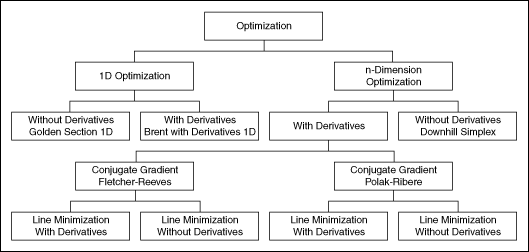# Optimization VIs

## LabVIEW 2018 Help

Edition Date: March 2018
Part Number: 371361R-01
View Product InfoLabVIEW 2016 HelpLabVIEW 2017 HelpLabVIEW 2018 HelpLabVIEW 2019 HelpLabVIEW 2020 Help

Owning Palette: Mathematics VIs

Requires: Full Development System. This topic might not match its corresponding palette in LabVIEW depending on your operating system, licensed product(s), and target.

Use the Optimization VIs to determine local minima and maxima of real 1D or n-dimension functions.

Example

You can choose between optimization algorithms based on derivatives of the function and algorithms working without these derivatives. You also can use special methods like Linear Programming, Levenberg-Marquardt in symbolic form, Pade, and Chebyshev Approximation.

The VIs on this palette can return mathematics error codes.

An overview of the optimization routines is shown in the following illustration.Palette ObjectDescription
Brent with Derivatives 1DDetermines a local minimum of a given 1D function in a given interval. The method is based on derivatives of the function.
Chebyshev ApproximationDetermines a given function using Chebyshev polynomials.
Conjugate Gradient nDDetermines a local minimum of a function of n independent variables with the Conjugate Gradient method.
Constrained Nonlinear OptimizationSolves a general nonlinear optimization problem with nonlinear equality constraint and nonlinear inequality constraint bounds using a sequential quadratic programming method.
Downhill Simplex nDDetermines a local minimum of a function of n independent variables with the Downhill Simplex method.
Find All Minima 1DDetermines all local minima of a given function in a given interval.
Find All Minima nDDetermines the minima of an n-dimension function in a given n-dimension interval.
Global OptimizationSolves a global optimization equation with boundary constraints, nonlinear equality constraints, and nonlinear inequality constraints using the differential evolution (DE) method.
Golden Section 1DDetermines a local minimum of a given 1D function with the help of a bracketing of the minimum. The Golden Section Search method is used.
Linear Programming Simplex MethodDetermines the solution of a linear programming problem.
Quadratic ProgrammingUses either an interior point algorithm or an active set algorithm to solve the problem: minimize 0.5x*Q*x + c*x, such that A*x=b and Imin are less than or equal to D*x, which is less than or equal to Imax. You must manually select the polymorphic instance to use.
Unconstrained OptimizationSolves the unconstrained minimization problem for an arbitrary nonlinear function. You must manually select the polymorphic instance to use.

## Example

Refer to the Transport Optimization Using Linear Programming VI in the labview\examples\Mathematics\Optimization directory for an example of using the Optimization VIs.